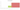# ل.س.‏

## Syrian Pound

Syria's official currency is the pound or lira. The Syrian Central Bank is the issuer. Despite the fact that piastre coins are no longer produced, the pound is divided into 100 of them. Prior to 1947, the word "qirsh" was written in Arabic with the first Arabic letter G, followed by the letter q. Arabic and French were the two languages used on the obverse and reverse of banknotes until 1958. The three designations for this coin are a result of the fact that English has been used on the reverses since 1958. French and Arabic were used on coins until the country gained its independence.

#### how has the value of the currency changed in the last year?## USD 1 = SYP 2,512.5

How much is10 US dollars worth inSyrian pounds?
At the current exchange rate, 10 US dollars is worth 25,125.3 Syrian pounds
How much is50 US dollars worth inSyrian pounds?
At the current exchange rate, 50 US dollars is worth 125,626.5 Syrian pounds
How much is100 US dollars worth inSyrian pounds?
At the current exchange rate, 100 US dollars is worth 251,253 Syrian pounds
How much is500 US dollars worth inSyrian pounds?
At the current exchange rate, 500 US dollars is worth 1,256,265 Syrian pounds
How much is2,000 US dollars worth inSyrian pounds?
At the current exchange rate, 2,000 US dollars is worth 5,025,060 Syrian pounds
How much is10 US dollars worth inSyrian pounds?
At the current exchange rate, 10 US dollars is worth 25,125.3 Syrian pounds
How much is50 US dollars worth inSyrian pounds?
At the current exchange rate, 50 US dollars is worth 125,626.5 Syrian pounds
How much is100 US dollars worth inSyrian pounds?
At the current exchange rate, 100 US dollars is worth 251,253 Syrian pounds
How much is500 US dollars worth inSyrian pounds?
At the current exchange rate, 500 US dollars is worth 1,256,265 Syrian pounds
How much is2,000 US dollars worth inSyrian pounds?
At the current exchange rate, 2,000 US dollars is worth 5,025,060 Syrian pounds